Deviant Art Shop Forum More Submit Join Login

## deviantIDaXforamnesty =a time for amnesty
United States
 "Be the change you want to see in the world"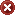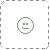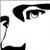Featured By Owner Dec 10, 2013   Digital Artist
Thank you for the fav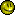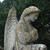Featured By Owner Dec 9, 2013  Hobbyist Traditional Artist
Thank you so much for the favs!  I really appreciate it!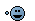P.S. Nice Gallery!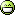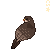Featured By Owner Sep 30, 2012  Professional Filmographer

I just finished Catching Fire, and I've been watching for the casting announcements! So excited, but it's not even started shooting yet. *sob*cloudylissa Featured By Owner Feb 6, 2011  Hobbyist General Artist
I <3 YOUUUUUUUUUUUUUU! YOU ARE TEH AWESOME FTW! <3<3<3<3<3<3<3<3<3<3<3<3<3<3<3<3<3<3<3<3<3<3<3<3<3<3<3<3<3<3<3<3<3<3<3<3<3<3<3<3<3<3<3<3<3<3<3<3<3<3<3<3<3<3<3<3<3<3<3<3<3<3<3<3<3<3<3<3<3<3<3<3<3<3<3<3<3<3<3<3<3<3<3<3<3<3<3<3<3<3<3<3<3<3<3<3<3<3<3<3<3<3<3<3<3<3<3<3<3<3<3<3<3<3<3<3<3<3<3<3<3<3<3<3<3<3<3<3<3<3<3<3<3<3<3<3<3<3<3<3<3<3<3<3<3<3<3<3<3<3<3<3<3<3<3<3<3<3<3<3<3<3<3<3<3<3<3<3<3<3<3<3<3<3<3<3<3<3<3<3<3<3<3<3<3<3<3<3<3<3<3<3<3<3<3<3<3<3<3<3<3<3<3<3<3<3<3<3<3<3<3<3<3<3<3<3<3<3<3<3<3<3<3<3<3<3<3<3<3<3<3<3<3<3<3<3<3<3<3<3<3<3<3<3<3<3<3<3<3<3<3<3<3<3<3<3<3<3<3<3<3<3<3<3<3<3<3<3<3<3<3<3<3<3<3<3<3<3<3<3<3<3<3<3<3<3<3<3<3<3<3<3<3<3<3<3<3<3<3<3<3<3<3<3<3<3<3<3<3<3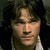Featured By Owner Jan 5, 2011
Thank you for the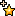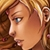Featured By Owner Jan 5, 2011
You bet!